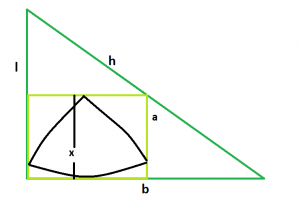# Biggest Reuleaux Triangle within a Square which is inscribed within a Right angle Triangle

Given here is a right angle triangle with height l, base b & hypotenuse h, which inscribes a square which in turn inscribes a reuleaux triangle. The task is to find the maximum possible area of this reuleaux triangle.

Examples:

```Input: l = 5, b = 12, h = 13
Output: 8.77914

Input: l = 3, b = 4, h = 5
Output: 2.07116
```## Recommended: Please try your approach on {IDE} first, before moving on to the solution.

Approach: We know, the side of the square inscribed within a right angled triangle is, a = (l*b)/(l+b), please refer Area of a largest square fit in a right angle triangle.
Also, in the reuleaux triangle, x = a.
So, x = (l*b)/(l+b).
So, Area of the Reuleaux Triangle is, A = 0.70477*x^2 = 0.70477*((l*b)/(l+b))^2.

Below is the implementation of the above approach:

## C++

 `// C++ Program to find the biggest Reuleaux triangle ` `// inscribed within in a square which in turn ` `// is inscribed within a circle ` `#include ` `using` `namespace` `std; ` ` `  `// Function to find the biggest reuleaux triangle ` `float` `Area(``float` `l, ``float` `b, ``float` `h) ` `{ ` ` `  `    ``// the height or base or hypotenuse ` `    ``// cannot be negative ` `    ``if` `(l < 0 || b < 0 || h < 0) ` `        ``return` `-1; ` ` `  `    ``// height of the reuleaux triangle ` `    ``float` `x = (l * b) / (l + b); ` ` `  `    ``// area of the reuleaux triangle ` `    ``float` `A = 0.70477 * ``pow``(x, 2); ` ` `  `    ``return` `A; ` `} ` ` `  `// Driver code ` `int` `main() ` `{ ` `    ``float` `l = 5, b = 12, h = 13; ` `    ``cout << Area(l, b, h) << endl; ` ` `  `    ``return` `0; ` `} `

## Java

 `// Java Program to find the biggest Reuleaux triangle ` `// inscribed within in a square which in turn ` `// is inscribed within a circle ` `import` `java.util.*; ` `import` `java.text.DecimalFormat; ` ` `  `class` `GFG ` `{ ` ` `  `// Function to find the biggest reuleaux triangle ` `static` `double` `Area(``double` `l, ``double` `b, ``double` `h) ` `{ ` ` `  `    ``// the height or base or hypotenuse ` `    ``// cannot be negative ` `    ``if` `(l < ``0` `|| b < ``0` `|| h < ``0``) ` `        ``return` `-``1``; ` ` `  `    ``// height of the reuleaux triangle ` `    ``double` `x = (l * b) / (l + b); ` ` `  `    ``// area of the reuleaux triangle ` `    ``double` `A = ``0.70477` `* Math.pow(x, ``2``); ` ` `  `    ``return` `A; ` `} ` ` `  `// Driver code ` `public` `static` `void` `main(String args[]) ` `{ ` `    ``double` `l = ``5``, b = ``12``, h = ``13``; ` `    ``DecimalFormat df = ``new` `DecimalFormat(``"#,###,##0.00000"``); ` `    ``System.out.println(df.format(Area(l, b, h))); ` `} ` `} ` ` `  `// This code is contributed by ` `// Shashank_Sharma `

## Python3

 `# Python3 Program to find the biggest  ` `# Reuleaux triangle inscribed within  ` `# in a square which in turn is inscribed ` `# within a circle ` `import` `math as mt  ` ` `  `# Function to find the biggest  ` `# reuleaux triangle ` `def` `Area(l, b, h): ` ` `  `    ``# the height or base or hypotenuse ` `    ``# cannot be negative ` `    ``if` `(l < ``0` `or` `b < ``0` `or` `h < ``0``): ` `        ``return` `-``1` ` `  `    ``# height of the reuleaux triangle ` `    ``x ``=` `(l ``*` `b) ``/``(l ``+` `b) ` ` `  `    ``# area of the reuleaux triangle ` `    ``A ``=` `0.70477` `*` `pow``(x, ``2``) ` ` `  `    ``return` `A ` ` `  `# Driver code ` `l, b, h ``=` `5``, ``12``, ``13` `print``(Area(l, b, h)) ` ` `  `# This code is contributed by ` `# Mohit kumar 29 `

## C#

 `// C# Program to find the biggest Reuleaux triangle ` `// inscribed within in a square which in turn ` `// is inscribed within a circle ` `using` `System; ` ` `  `class` `GFG ` `{ ` ` `  `// Function to find the biggest reuleaux triangle ` `static` `double` `Area(``double` `l, ``double` `b, ``double` `h) ` `{ ` ` `  `    ``// the height or base or hypotenuse ` `    ``// cannot be negative ` `    ``if` `(l < 0 || b < 0 || h < 0) ` `        ``return` `-1; ` ` `  `    ``// height of the reuleaux triangle ` `    ``double` `x = (l * b) / (l + b); ` ` `  `    ``// area of the reuleaux triangle ` `    ``double` `A = 0.70477 * Math.Pow(x, 2); ` ` `  `    ``return` `A; ` `} ` ` `  `// Driver code ` `public` `static` `void` `Main() ` `{ ` `    ``double` `l = 5, b = 12, h = 13; ` `    ``Console.WriteLine((Area(l, b, h))); ` `} ` `} ` ` `  `// This code is contributed by ` `// Mukul Singh `

## PHP

 ` `

Output:

```8.77914
```

My Personal Notes arrow_drop_upCheck out this Author's contributed articles.

If you like GeeksforGeeks and would like to contribute, you can also write an article using contribute.geeksforgeeks.org or mail your article to contribute@geeksforgeeks.org. See your article appearing on the GeeksforGeeks main page and help other Geeks.

Please Improve this article if you find anything incorrect by clicking on the "Improve Article" button below.